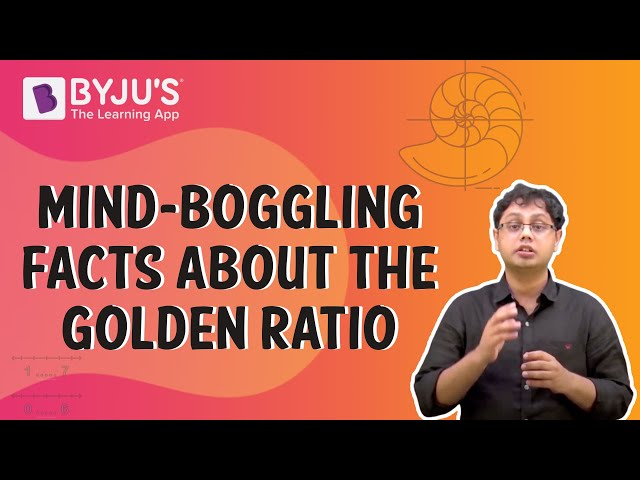# Explain about the golden ratio?

A golden ratio is an approximately equivalent number to 1.618. Sometimes it occurs in geometry, architecture, art and other sectors. If their ratio is the same as the ratio of their sum to the larger of the two quantities then it is the golden ratio.

The Golden Ratio is a specific number identified by splitting a line into two sections such that the longer part divided by the smaller part is equivalent to the total length divided by the longer part.

The golden ratio is denoted by the Greek alphabet phi ( ).

a/b = (a+b)/a = 1.6180339887498948420 …

is generally rounded to 1.618. That number has been found and rediscovered several times, that’s why it has many other versions of terms such as the Golden section, Golden mean, divine proportion, and so on.

It has been said that two numbers are in the golden ratio if the ratio of their sum to the larger number is equal to the ratio of the greater number to the smaller number. So if a & b are in the golden ratio then

(a+b)/a = a/b =

### Fibonacci number Serice

The golden ratio is also closely related to the Fibonacci series. The special properties of this sequence were discovered by the mathematician Leonardo Fibonacci during 1200.

The sequence begins from 0, 1, 1, 2, 3, 5, 8, 13, 21, 34, 55, 89, 144 and so on. This magical series is directly related to the Golden Ratio since if you take any two consecutive numbers from Fibonacci, their ratio is very similar to the Golden Ratio. As the numbers go up, the ratio gets much closer to 1,625.(8)(0)